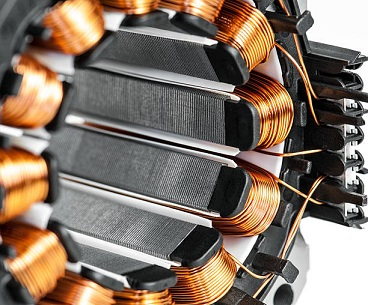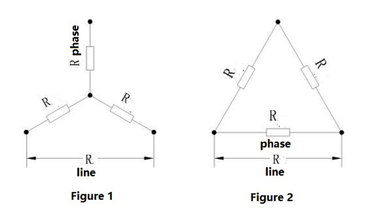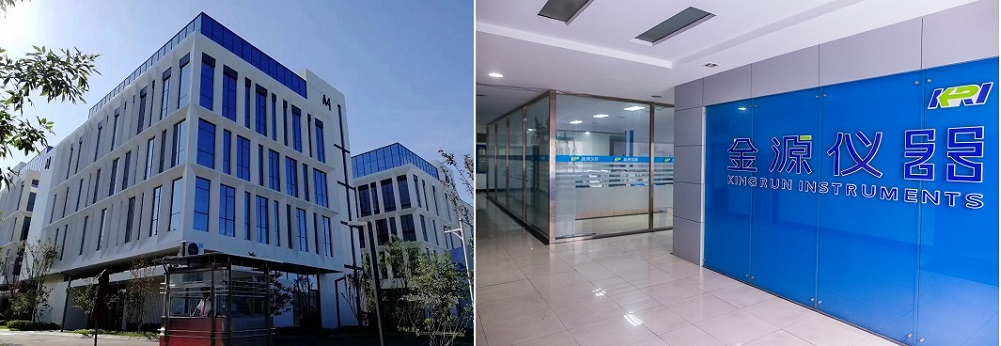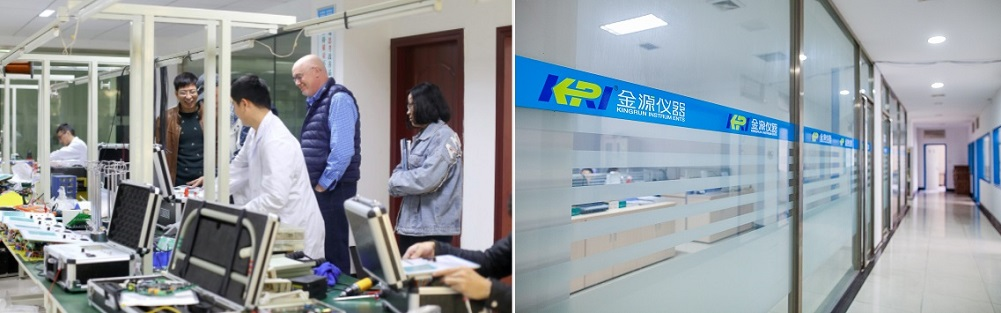News

# How to Convert between Line Resistance and Phase Resistance

What is the DC winding resistance, and how is it tested?

DC resistance is an important parameter of motor or transformer windings, it is related to many factors such as the design of the motor or transformer winding, the material of the magnet wire used, and the ambient temperature. In the process of motor and transformer inspection test and type test, DC resistance detection is a must-tested item; for motor and transformer companies that standardize production, DC resistance detection will be carried out before the winding iron core is dipped in paint, so as to avoid bad product enters the subsequent production link.Determination of DC resistance is also an important part of the motor or transformer test. Through the analysis of the measured resistance value, it can be preliminarily determined whether the number of turns, wire diameter, number of parallel windings, wiring method and wiring quality of the tested windings meet the requirements, and whether there is a serious short-circuit fault between the winding turns. The DC resistance of the winding is involved in the calculation of motor or transformer loss and temperature rise, which directly affects the effect of motor or transformer performance evaluation. Therefore, the measurement test of the DC resistance of the winding should choose a test instrument with a higher precision, and strive to have a higher accuracy of the detection data.

When measuring the DC resistance of the winding in the motor or transformer test, the DC resistance measuring instrument (such as resistance tester, DC bridge, etc.) is generally used for direct measurement, and sometimes the voltage and current methods are used (generally not used).If the phase resistance of the winding is R phase and the line resistance is R line, the calculation of the line resistance can be calculated according to the series-parallel principle of the resistor.

●Line resistance by phase resistance

When the motor winding is star-connected, the schematic is shown in Figure 1, and the R line = 2R phase.

When the motor winding is at the corner, the schematic is shown in Figure 2, and the R line = 2R phase / 3.

●The phase resistance is converted by line resistance

The formula for calculating the line resistance from the phase resistance can be mathematically converted under different connections.

When the motor winding is star-connected, the schematic is shown in Figure 1, R phase = 0.5R line

When the motor winding is angled, the schematic is shown in Figure 2, and the R phase = 1.5R line.● Conversion of resistance values at different temperatures

Generally, the DC resistance of a metal conductor has a fixed relationship with its temperature. This relationship is expressed as:

R1=R2×(K+t1)/(K+t2).........(1)

Where: R1 - DC resistance (Ω) at a temperature of t1 ° C;

R2——DC resistance (Ω) at a temperature of t2°C;

K - constant (reciprocal of the temperature coefficient of the conductor resistance at 0 ° C), for copper windings, K = 235, for aluminum windings, K = 225.

● Resistance standard under different test conditions

In the type test report, the specific resistance value is given according to the different insulation grade of the motor, such as the resistance of the B-class insulated motor at 75 °C, while the F-class insulated motor is the resistance at 95 °C, and the H-class insulated motor is Resistance at 105 °C. In the inspection test evaluation standard, it is customary to give the resistance at 20 ° C. When the temperature deviates, it can be converted according to the formula (1).

is suitable for small distribution transformer (below 80KVA) it is provided with the temperature conversion function. By selecting test sample material(copper/aluminum) and temperature from tester, the instrument can be able to automatically convert them to the resistance values under the standard temperature (20℃,75℃,120℃). KRI 9310 is also provided with the data storage function, 500 groups of experimental data can be stored within the instrument. also KRI 9310 has USB interface, the data can be down load to flash disc.

Related Articles:

DC winding resistance tester with 500 times tests on a single charge -JYR9310

Please Click below Pictures for More KINGRUN DC Winding Resistance Testers (Output Current from 5~50A):

All Kingrun Testers will be Delivered by International Air Express for Free!